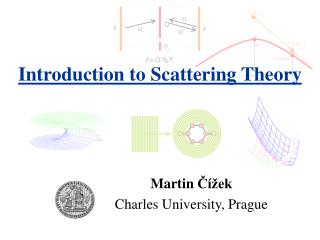DownloadDownload PresentationIntroduction to Scattering Theory

# Introduction to Scattering Theory

Télécharger la présentation## Introduction to Scattering Theory

- - - - - - - - - - - - - - - - - - - - - - - - - - - E N D - - - - - - - - - - - - - - - - - - - - - - - - - - -
##### Presentation Transcript

1. R H H B H=RB t = 0 t > 0 Introduction to Scattering Theory Martin Čížek Charles University, Prague

2. Formal Scattering Theory - Intro Time evolution: Formal solution: t = 0 t > 0

3. Formal Theory: Asymptotes Hamiltonian: Definition: in-asymptote t = 0 t < 0

4. Formal Theory: Asymptotes Definition: out-asymptote t = 0 t > 0

5. Asymptotic condition The theory is said to fulfill the asymptotic condition if for every exists for which: + the same for t → +∞ Møller wave operators:

6. Asymptotic completeness The theory is said to be asymptoticly complete if Ω+(H) = Ω-(H) = R (orthogonal complement toB) R H H B H=RB

7. Scattering operator Unitarity: S+ S = S S+=1 R H H B In asymptotes Out asymptotes

8. Energy conservation Intertwining relations: Corollary i.e. we can define “On-Shell T-matrix” S 1 + remainder

9. The Cross Section

10. The Cross Section It can be shown, that the procedure does not depend on the shape of ψin=φ(p), provided φ(p) is sharply peaked around p0 Key assumption is

11. Symmetries: Rotational Invariance Rotation described by Rotational invariance: [H,R(α)] = [H0, R(α)] = 0 Consequence: [R(α),Ω±] = [R(α),S] = 0 S-matrix is diagonal in basis formed by common eigenvectors of operators H0, J2, J3

12. Symmetries: Rotational Invariance

13. More on symmetries Parity invariance: Time reversal:

14. Resolvent – Green’s operator Significance: 1. Green’s function for time-independent Schrodinger equation 2. Fourier transform of evolution operator Resolvent (Lippmann-Schwinger) equation: G=G0+G0VG

15. Green and Møller It is possible to show: i. e.

16. Green’s and T-operator Lippmann-Schwinger for T: Born series

17. Scattering and T-operators Recall:

18. Stationary scattering states Recall: We apply: Lippmann-Schwinger eqution Spatial representation:

19. Partial wave expansions Free wave (just question to expand |p in terms of |E,l,m) Stationary scattering state: Note:

20. Partial waves – integral eqation

21. Normalized / Regular solution Jost function

22. Analytic properties • φl,p(r) is analytic function of p, λ • Jost function is analytic in λ • Jost function is analytic in p in upper half-plain • Zeros of Jost function = poles of s-matrix: physical sheet Im p bound states Re p virtual state resonances

23. Rieman surface for the energy E=p2/2m Re E

24. S, T, K - matrix Recall: (1-S) and 2πiT was the same “on shell” more generally T(z)=V+VG(z)V Def: M=i(1-S)(1+S)-1 i. e. S=(1+iM)(1-iM)-1 K-matrix (reaction or Heitler’s matrix): note: it is possible to define it in analogy for T with standing-wave G

25. Literature • J. R. Taylor: Scattering Theory, • R. G. Newton: Scattering Theory of Waves and Particles, • P. G. Burke: Potential Scattering in Atomic Physics